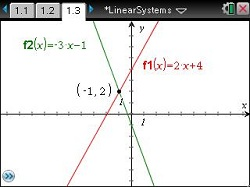# Activities

••• ##### Subject Area

• Math: Algebra II: Equations
• Math: Algebra II: Systems of Linear Equations and Inequalities
• Math: College Algebra: Linear Systems and Matrices

• ##### Author9-12

15 Minutes

• ##### Device
•TI-Nspire™ CX/CX II
•TI-Nspire™ CX CAS/CX II CAS
• TI-Nspire™
• TI-Nspire™ CAS
• ##### Software

TI-Nspire™
TI-Nspire™ CAS

3.0

## Intersecting the Solutions#### Activity Overview

In this teacher-led activity, students will learn to solve systems of equations graphically. They will learn the relationship between the algebraic and graphical solutions and create equations that draw upon this connection.

#### Key Steps

•In this problem, students will solve a system of linear equations algebraically, using substitution, and solving it graphically on the calculator. Students will see the relationship between the algebraic solution and the graphical solution.

Through solving the systems algebraically, they should discover that the values of x and y are the point of intersection (x, y) when the two lines in the system are graphed.